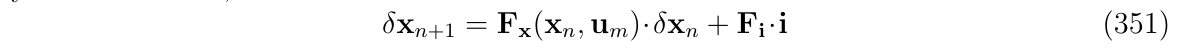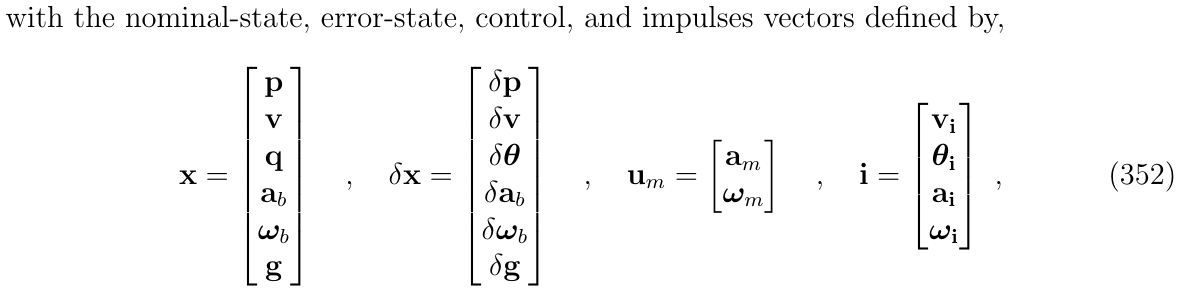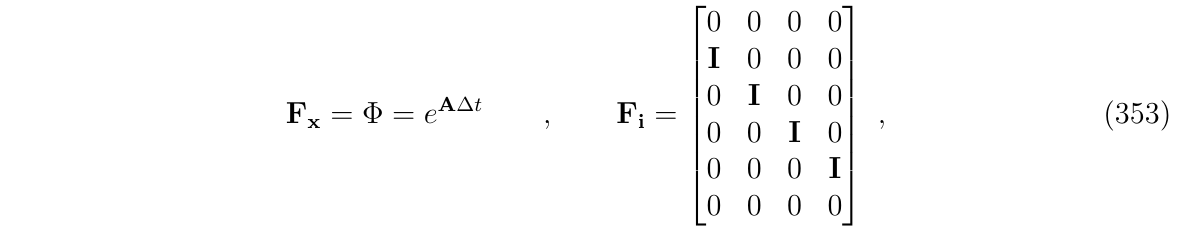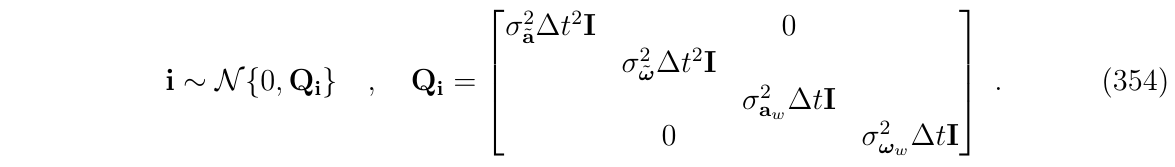# 对随机噪声和干扰的积分处理

$\dot{x}=f(x,u,w)$

• $$x$$是状态向量
• $$u$$是控制信号，含有噪声$$\tilde{u}$$，因此，对控制量的观测$$u_m=u+\tilde{u}$$
• $$w$$是随机干扰的向量

$\tilde{u} \sim \mathcal{N}\{0,U^c\}$

$w^c \sim \mathcal{N} \{0, W^c\}$

• 离散化的时候，控制信号在固定时间$$n\Delta t$$被采样，于是有$$u_{m,n}\triangleq u_m(n\Delta t)=u(n\Delta t)+\tilde{u}(n \Delta t)$$，测量部分在积分间隔内被看做是固定的常数，如$$u_m(t)=u_{m,b}$$，因此，在采样时间$$n\Delta t$$时刻的噪声也被认为是常数，即

$\tilde{u}(t)=\tilde{u}(n\Delta t)=\tilde{u}_n,~~ n\Delta t < t <(n+1) \Delta t \tag{324}$

• 而扰动项$$w$$不能被采样

## 标准形式处理噪声和扰动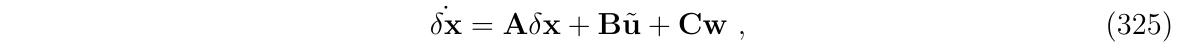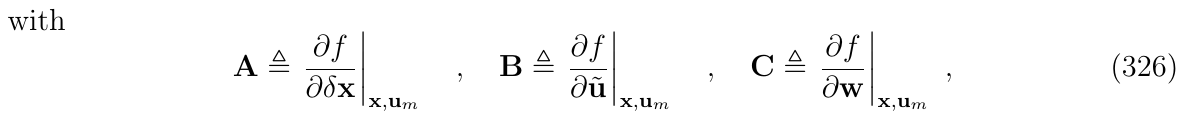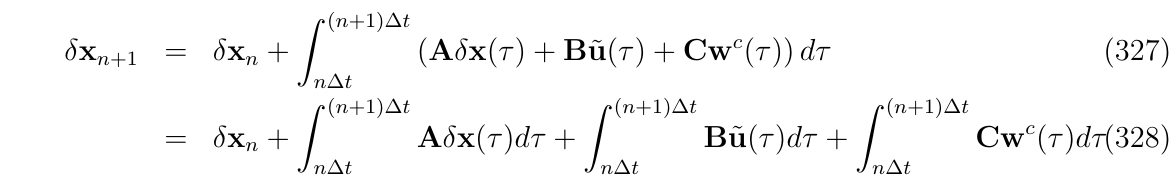• 状态转移部分: 从附录B，我们知道动态的部分是积分得到的转移矩阵，即: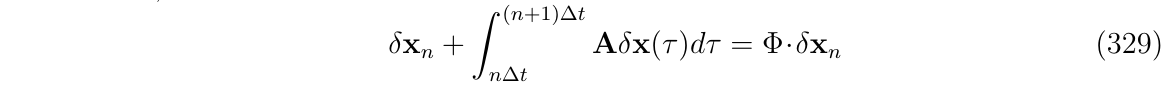• 测量噪声部分: 从式(324)，可以得到：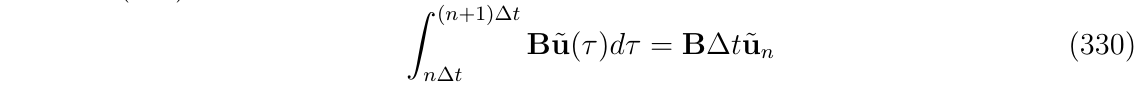• 随机干扰部分: 从概率论，我们知道在时间间隔$$\Delta t$$内对连续时间的高斯白噪声进行积分，将产生离散的高斯脉冲$$w_n$$，如下: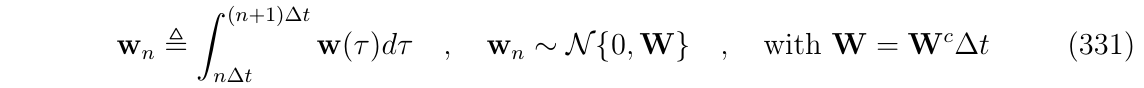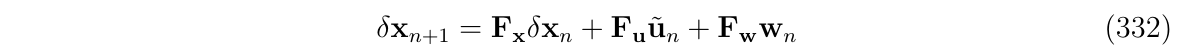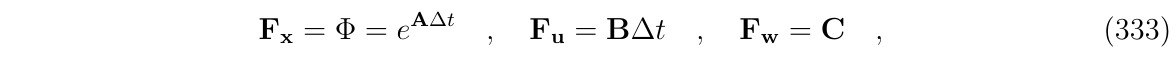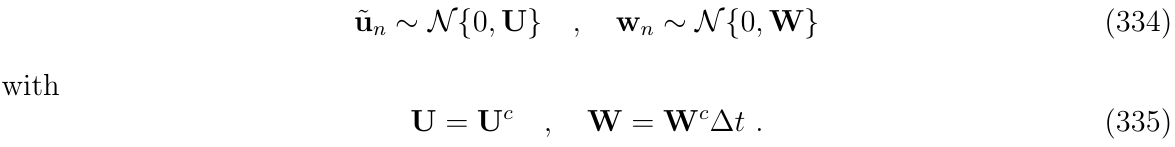## 总结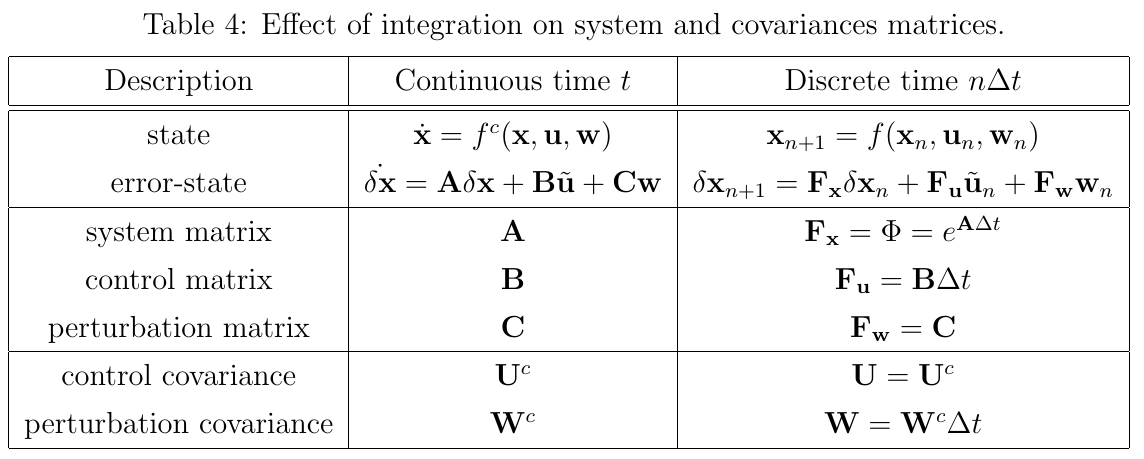EKF的预测阶段，将会传播误差状态的均值和方差，根据如下方程: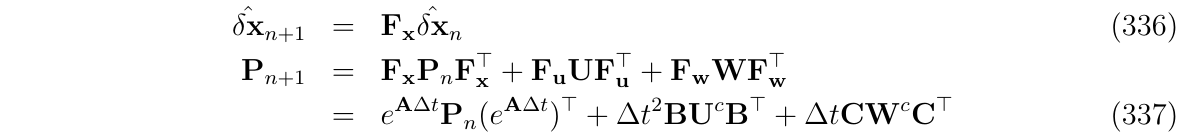• 动态误差项是指数函数形式的$$e^{A \Delta t}$$
• 测量噪声项是二次的
• 干扰误差项是线性的(一次)

# 简化形式处理噪声和扰动脉冲

## 简化形式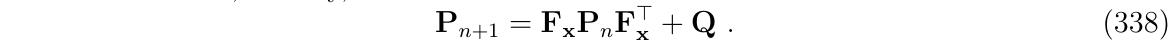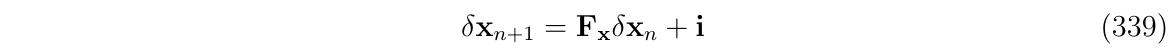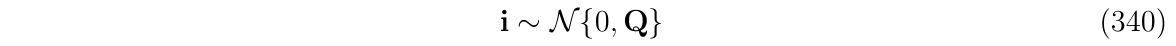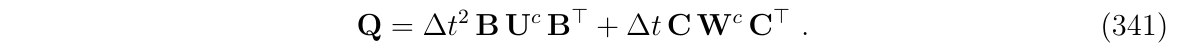## 进一步

(式339)可以变成: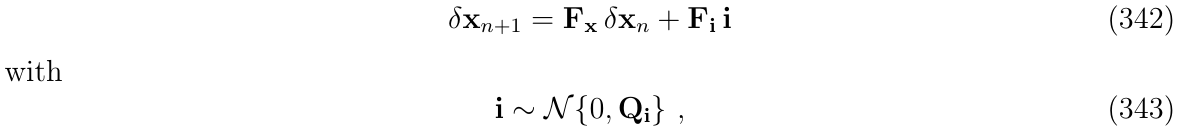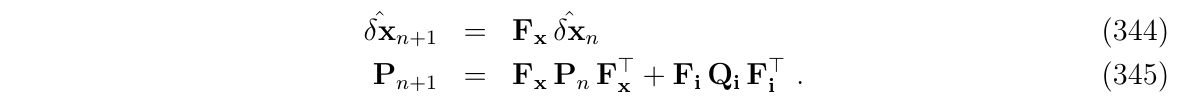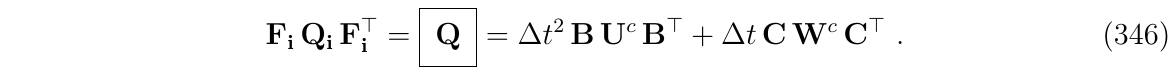# 完整版IMU例子

## 使用标准形式处理噪声和扰动

• nominal state x，
• error-state $$\delta x$$
• 控制信号$$u_m=u+\tilde{u}$$
• 干扰$$w$$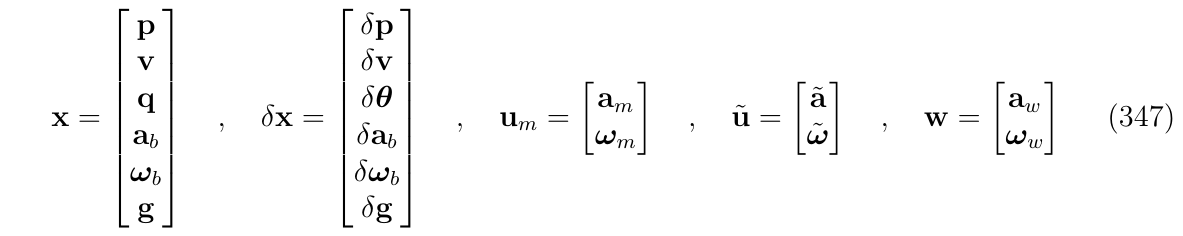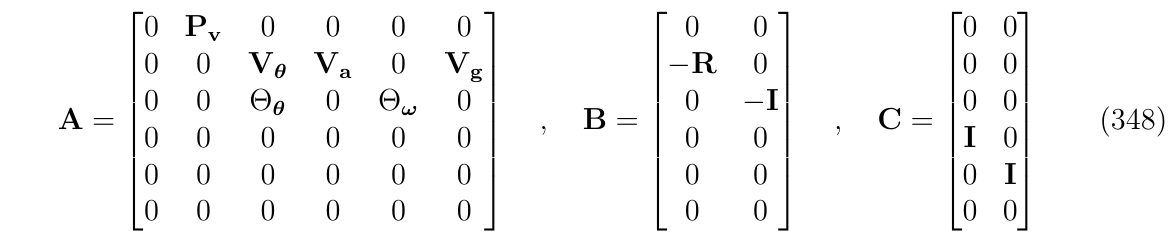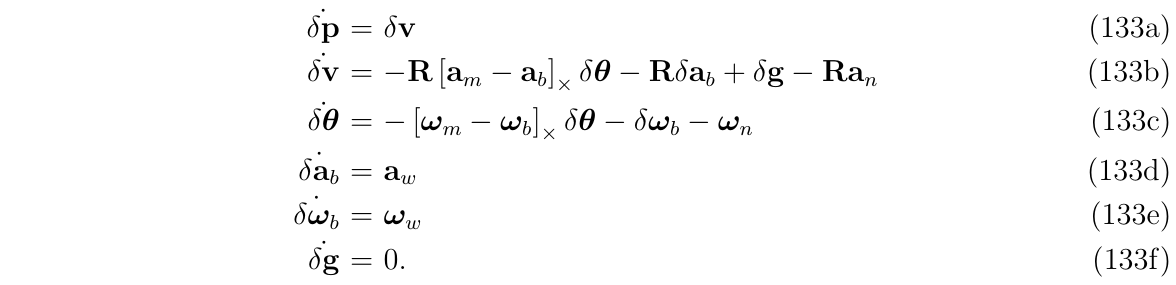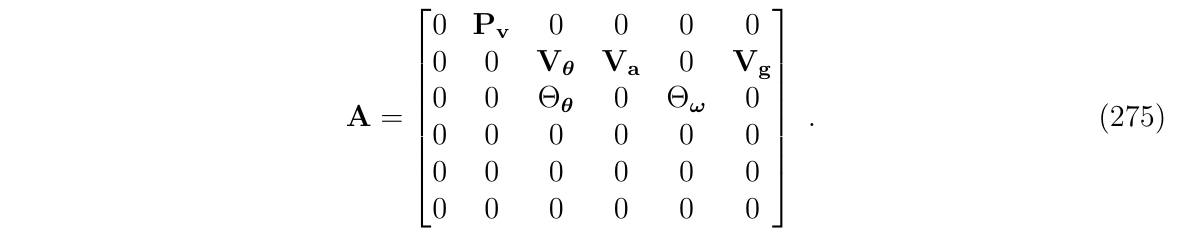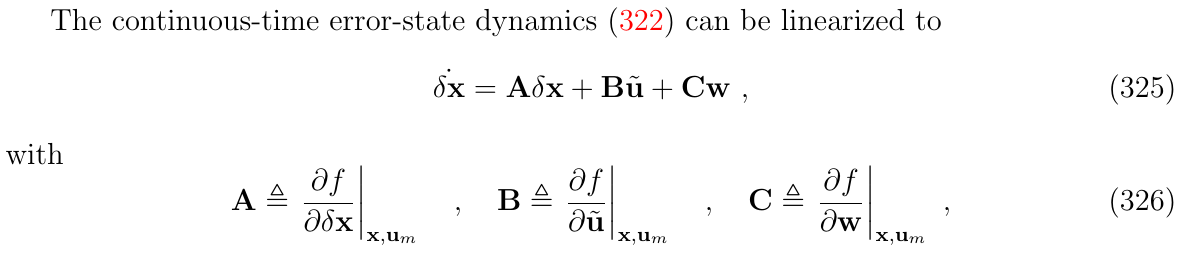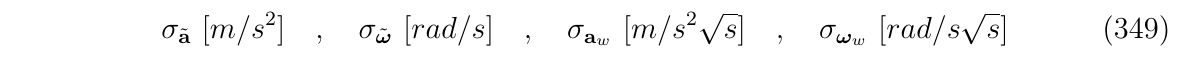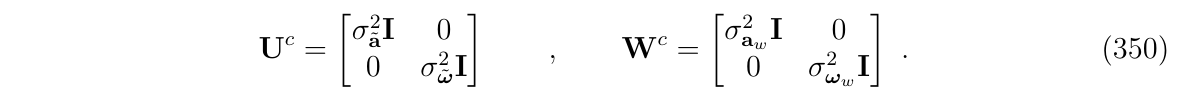## 使用简化形式处理噪声和扰动(常见)